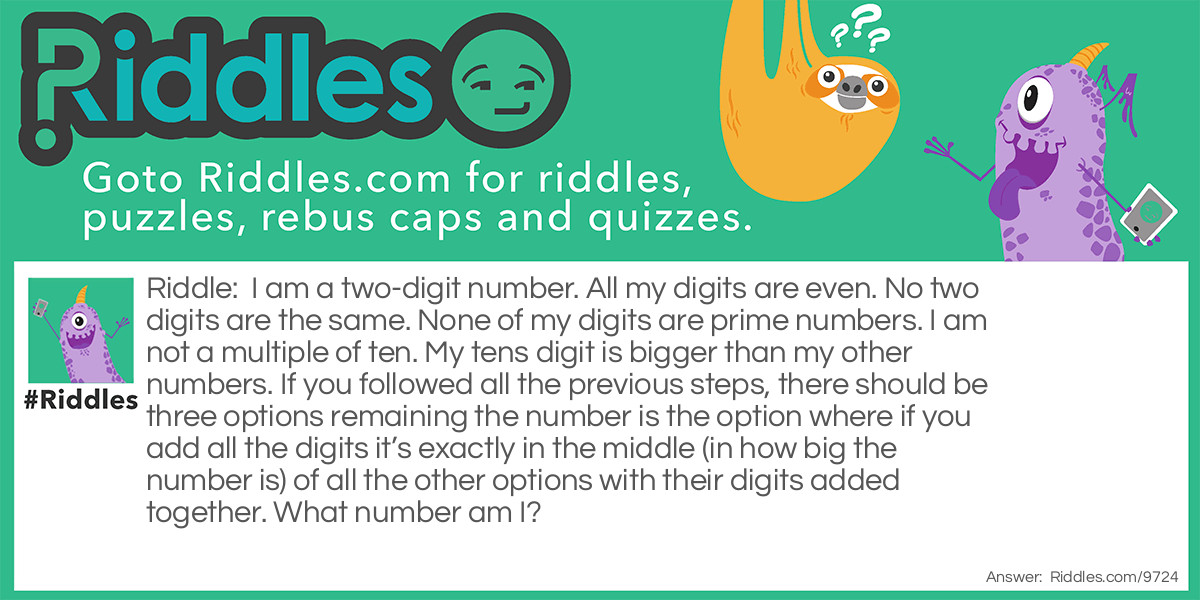ADVERTISEMENT
Riddle:

I am a two-digit number. All my digits are even. No two digits are the same. None of my digits are prime numbers. I am not a multiple of ten. My tens digit is bigger than my other numbers. If you followed all the previous steps, there should be three options remaining the number is the option where if you add all the digits it’s exactly in the middle (in how big the number is) of all the other options with their digits added together. What number am I?

Answer:

If you followed all the steps apart from the last one there will be three options remaining: 64, 84, and 86. You then had to add up the digits, 64=6+4=10, 84=8+4=12, and 86=8+6=14. Finally, you then had to take up the middle biggest number (12) and put it back as it was before the digits were added together and your answer should be 84.

VOTE
SHARE
COMMENTThe even number. Riddle Meme with riddle and answer link.
ADVERTISEMENT